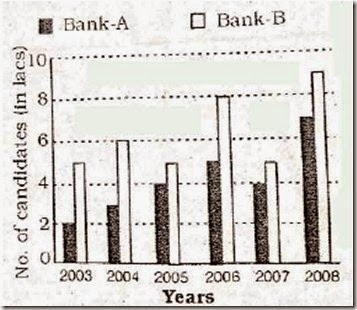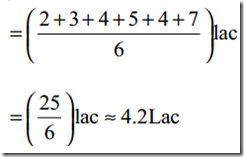Wednesday, 29 April 2015

Friends Take A Practice Now with Previously Asked Questions In bank Po Exam  and See last Pos On SBI PO Data Interpretation test –5 With Solution (BAR GRAPHS)

Directions(1-5): Study the following  graph carefully to answer the questions that

follow.

Number of Girls and Boys Participating in a Rally From Five Different Schools

1. What is the total number of girls participating in the rally from schools A and

C together?

1) 825 2) 875 3) 950

4) 975 5) None of these

2. The number of boys participating the rally school B is what percent of the total number  of children participating in the rally from that school? (Rounded off two digits after decimal)

1) 48.84 2) 47.37 3) 49.28

4) 46.46 5) None of these

3. The number of boys participating the rally school E is approximately what percent of the number of boys participating in the rally from the same school?

1) 81 2) 106 3) 122 4) 98 5) 114

4. What is the respective ratio of total number of girls participating in the rally from school D and E together to the total number of boys participating in the rally from school A and B together?

1) 23 : 18 2) 43 : 35 3) 41 : 38

4) 21 : 20 5) None of these

5. What is the average number of girls participating in the rally from school together?

1) 500 2) 480 3) 525

4) 495 5) None of these

Directions (6-10): Study the following graph carefully to answer the questions that follow.

Number of applicants ( in lacs ) who applied for two different banks during past six years6. What is the approximate average number of candidates who applied for Bank-A over all the years together?

1) 4.2 lacs 2) 5 lacs 3) 3.1 lacs

4) 4.5 lacs 5) 3.8 lacs

7. Total number of candidates who applied for Bank-A over all the years together was approximately what percentage of the total number the candidates who applied for Bank-B over all the years together?

1) 79 2) 66 3) 70

4) 75 5) 60

8. In which years the total number of candidates who applied for both the banks together is exactly equal?

1) 2004, 2005 and 2008

2) 2004, 2006 and 2007

3) 2004, 2005 and 2007

4) 2004, 2006 and 2008

5) 2006, 2007 and 2009

9. If 20 percent of who applied for Bank-B qualified in the year 2008, then what was the number of candidates who have been disqualified in the same year?

1) 7.2 lacs 2) 72,000 3) 6.4 lacs

4) 64,000 5) None of these

10. What was the respective ratio between the total number of candidates who applied for Bank A in the year 2006 and 2008 and the total number of candidates who applied for Bank-B in the year 2005 and 2008 together?

1) 6 : 7 2) 4 : 9 3) 5 : 12

4) 12 : 5 5) None of these

1. (5)

Required number of girls = 450 + 475 = 925

2. (1)

3 (3)

4. (4) Required ratio = (500 + 550) : (475 + 525)
= 1050 : 1000
= 21 : 20

5. (5) Required average number of girls

= (450+550+475+500+550)/5 = 2525/5  =505

6. (1)
Required average7. (2)
Number of candidates over all the years:
Bank A⇒25 lac
Bank B ⇒ (5 + 6 + 5 + 8 + 5 + 9) lac
= 38 lac
Required percentage  = (25/38)x100 =66

8. (3)
It is obvious from the graph

9. (1)
Required number of candidates who have
been disqualified

=(9x80/100)lac = 7.2 lac

10. (1)
Required ratio = 12 : 14 = 6 : 7